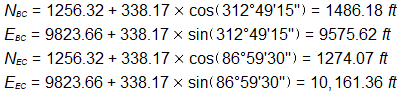#### Problem (1)

Given the following information:Δ = 45°49'45"
D = 07°09'43.1"
L = 693.90 ft
T = 338.17 ft
LC = 622.97 ft

Curve Table

 Station di, ft δi ci, ft EC Back 57+24.88 639.90 = L 24°54'52" = Δ/2 622.97 = LC 57+00 615.20 22°01'26" 599.99 56+00 515.02 18°26'34" 506.18 55+00 415.02 14°51'43" 410.38 54+00 315.02 11°16'51" 312.99 53+00 215.02 7°42'00" 214.38 52+00 115.02 4°07'08" 114.92 51+00 15.02 0°32'17" 15.02 BC 50+84.98 0.000 0°00'00" 0.000

##### Determine curve point coordinates.

Compute azimuth of incoming tangentCompute coordinates of BC and EC

####Set up the azimuth and coordinates equations

####Using the az and coord equations, step through the table for each curve point

 Station Azi Ni, ft Ei, ft EC Back 57+24.88 107°54'23" 1274.07 check 10,161.38 check 57+00 110°47'26" 1273.15 10,136.51 56+00 114°22'40" 1277.25 10,036.66 55+00 117°57'32" 1293.78 9,938.10 54+00 121°32'24" 1322.46 9,842.37 53+00 125°07'15" 1362.85 9,750.96 52+00 128°46'07" 1414.32 9,665.30 51+00 132°16'58" 1584.39 9,834.78 BC 50+84.98 132°49'15" 1486.18 9,575.62

#### Problem (2)

For the following short alignment data:##### What are the coordinates of curve station 20+00.00?

NPI = 1151.53 ft
EPI = 1131.30 ft
NBC = 1052.74 ft
EBC = 589.35 ft
δ20+00 = 14°07'03"
c20+00 = 698.76 ft
Az20+00 = 93°47'13"
N20+00 = 1006.59 ft
E20+00 = 1286.58 ft